Скачать презентацию Risk Analysis Risk generally refers to outcomes that

• Количество слайдов: 24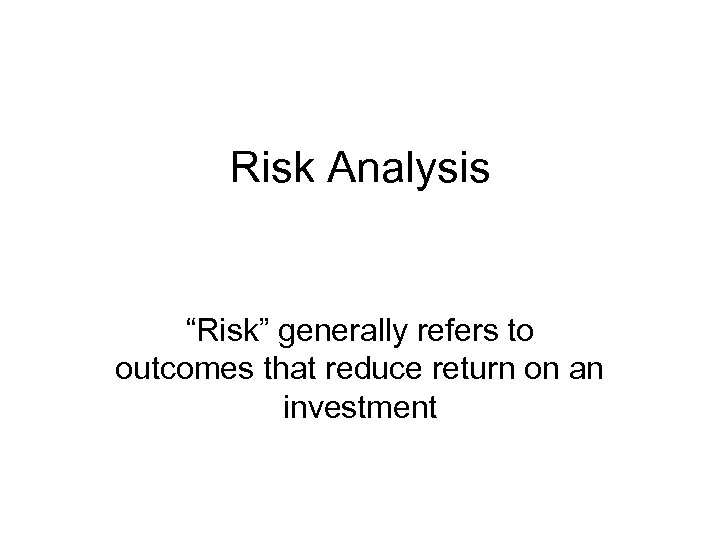Risk Analysis “Risk” generally refers to outcomes that reduce return on an investment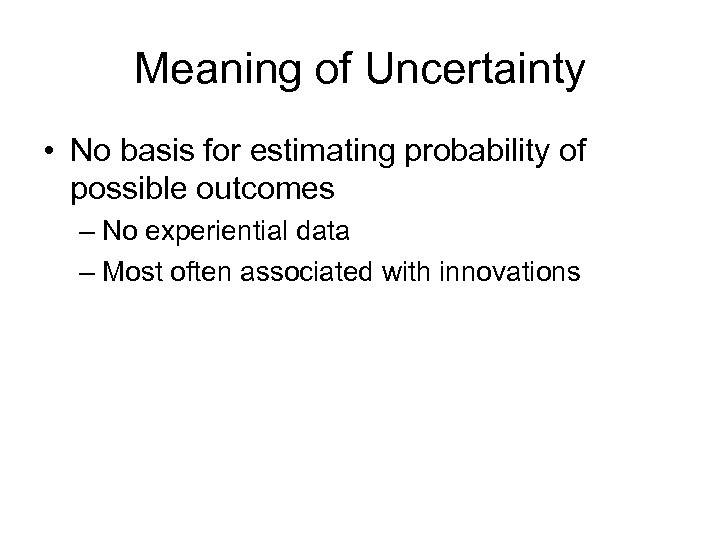Meaning of Uncertainty • No basis for estimating probability of possible outcomes – No experiential data – Most often associated with innovations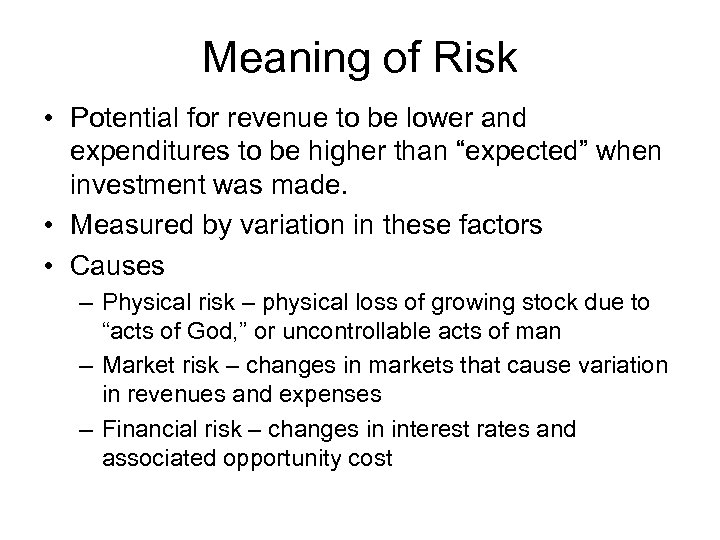Meaning of Risk • Potential for revenue to be lower and expenditures to be higher than “expected” when investment was made. • Measured by variation in these factors • Causes – Physical risk – physical loss of growing stock due to “acts of God, ” or uncontrollable acts of man – Market risk – changes in markets that cause variation in revenues and expenses – Financial risk – changes in interest rates and associated opportunity cost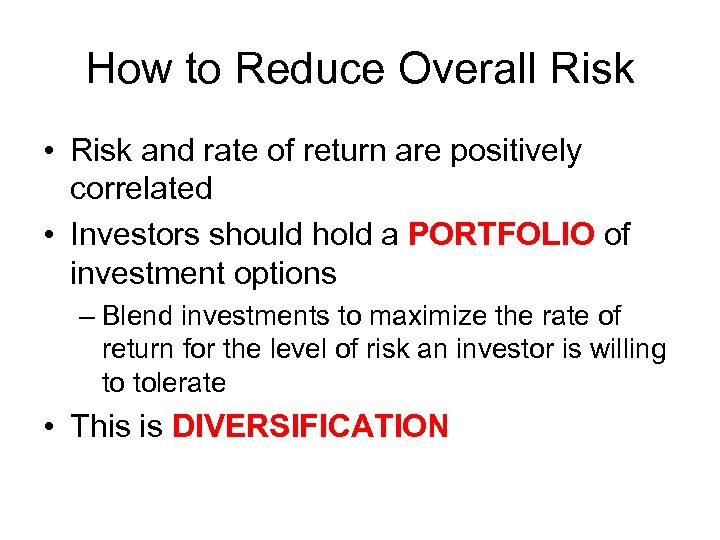How to Reduce Overall Risk • Risk and rate of return are positively correlated • Investors should hold a PORTFOLIO of investment options – Blend investments to maximize the rate of return for the level of risk an investor is willing to tolerate • This is DIVERSIFICATION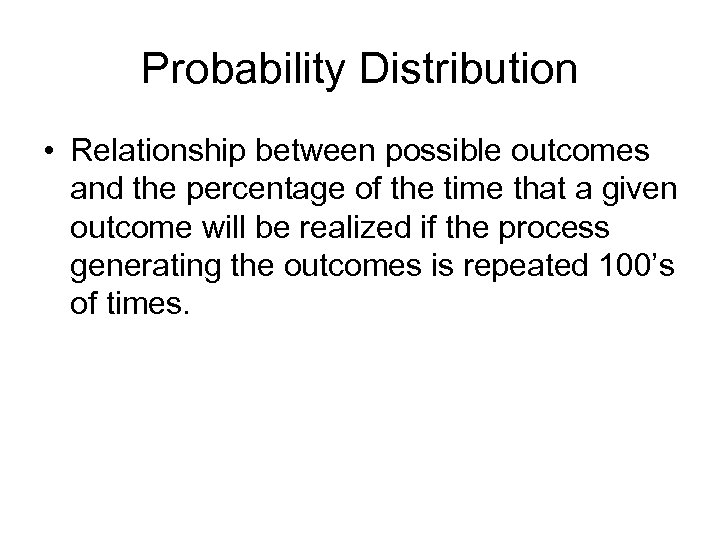Probability Distribution • Relationship between possible outcomes and the percentage of the time that a given outcome will be realized if the process generating the outcomes is repeated 100’s of times.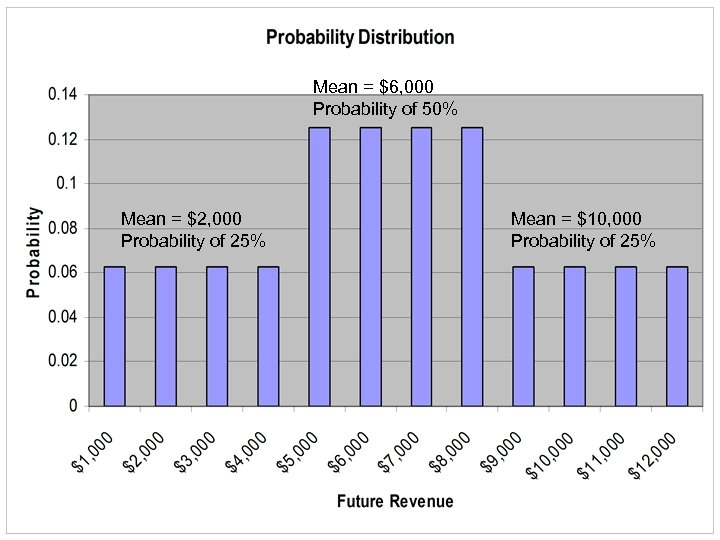Mean = \$6, 000 Probability of 50% Mean = \$2, 000 Probability of 25% Mean = \$10, 000 Probability of 25%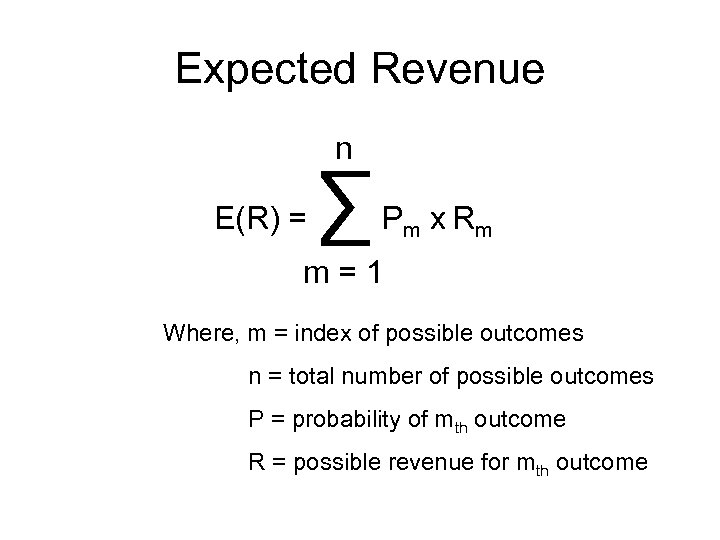Expected Revenue n E(R) = ∑ P m x Rm m=1 Where, m = index of possible outcomes n = total number of possible outcomes P = probability of mth outcome R = possible revenue for mth outcome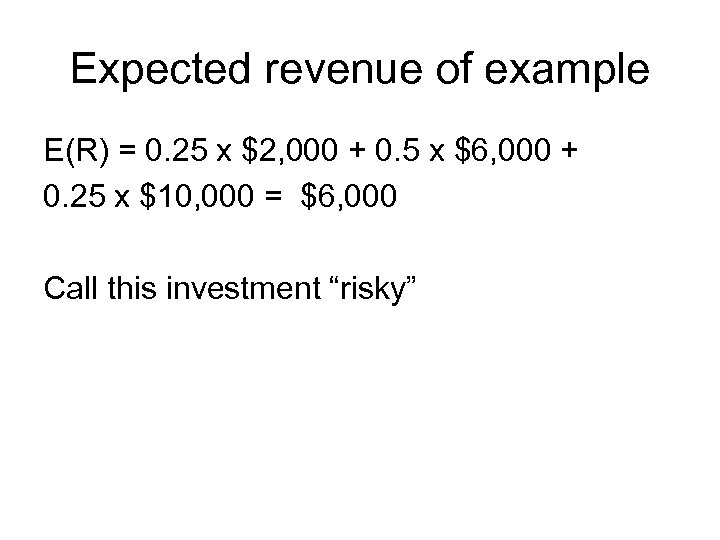Expected revenue of example E(R) = 0. 25 x \$2, 000 + 0. 5 x \$6, 000 + 0. 25 x \$10, 000 = \$6, 000 Call this investment “risky”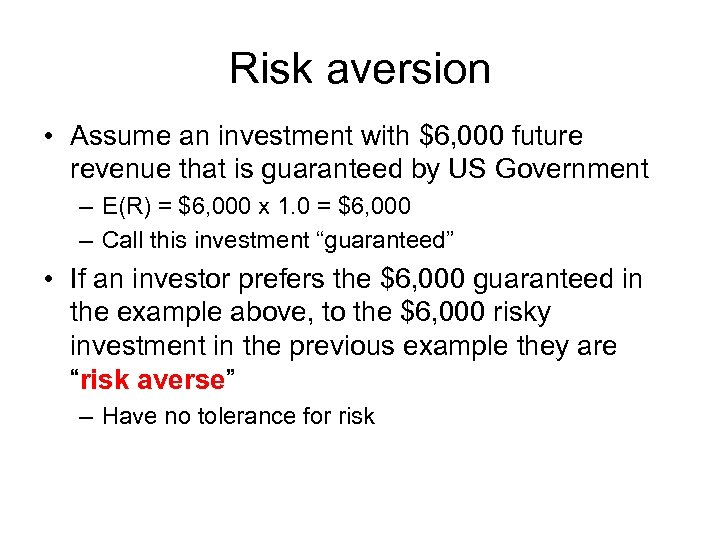Risk aversion • Assume an investment with \$6, 000 future revenue that is guaranteed by US Government – E(R) = \$6, 000 x 1. 0 = \$6, 000 – Call this investment “guaranteed” • If an investor prefers the \$6, 000 guaranteed in the example above, to the \$6, 000 risky investment in the previous example they are “risk averse” – Have no tolerance for risk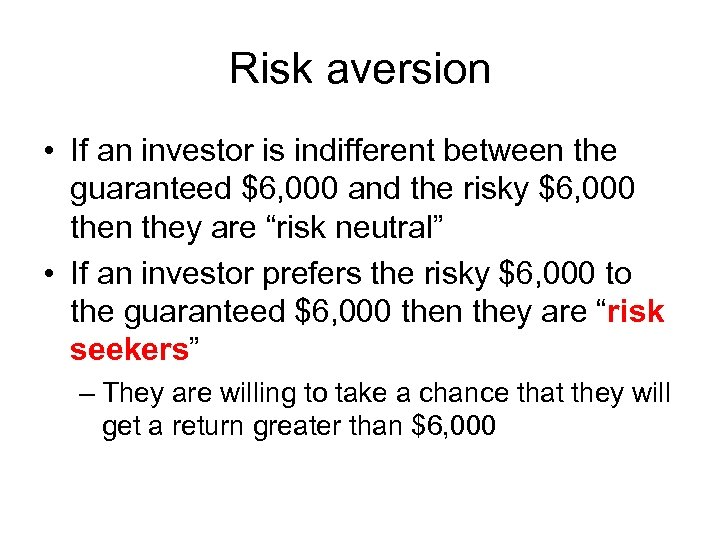Risk aversion • If an investor is indifferent between the guaranteed \$6, 000 and the risky \$6, 000 then they are “risk neutral” • If an investor prefers the risky \$6, 000 to the guaranteed \$6, 000 then they are “risk seekers” – They are willing to take a chance that they will get a return greater than \$6, 000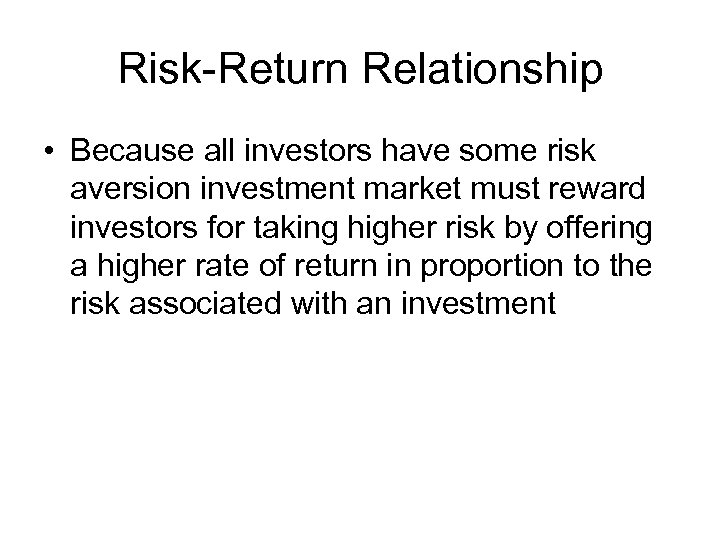Risk-Return Relationship • Because all investors have some risk aversion investment market must reward investors for taking higher risk by offering a higher rate of return in proportion to the risk associated with an investmentVariation • Sum of squared deviations from expected revenue weighted by probability of outcome n • Variance = σ2 = ∑ [R – E(R)]2 x Pm m m=1 • Standard deviation = (σ2 )1/2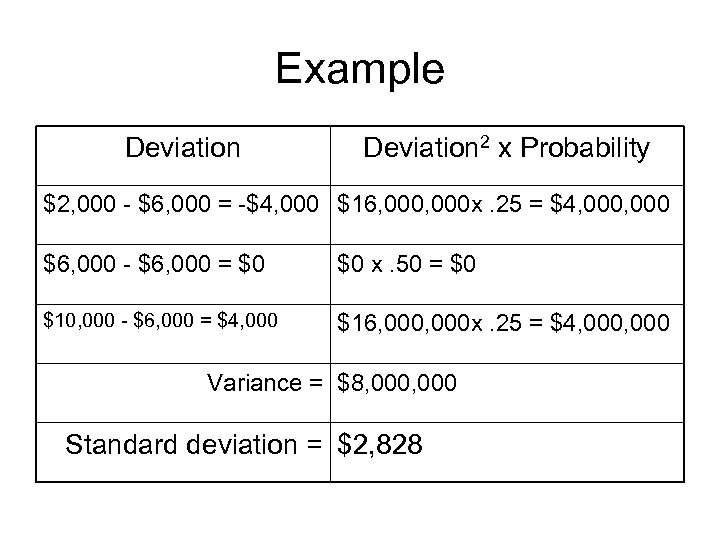Example Deviation 2 x Probability \$2, 000 - \$6, 000 = -\$4, 000 \$16, 000 x. 25 = \$4, 000 \$6, 000 - \$6, 000 = \$0 \$0 x. 50 = \$0 \$10, 000 - \$6, 000 = \$4, 000 \$16, 000 x. 25 = \$4, 000 Variance = \$8, 000 Standard deviation = \$2, 828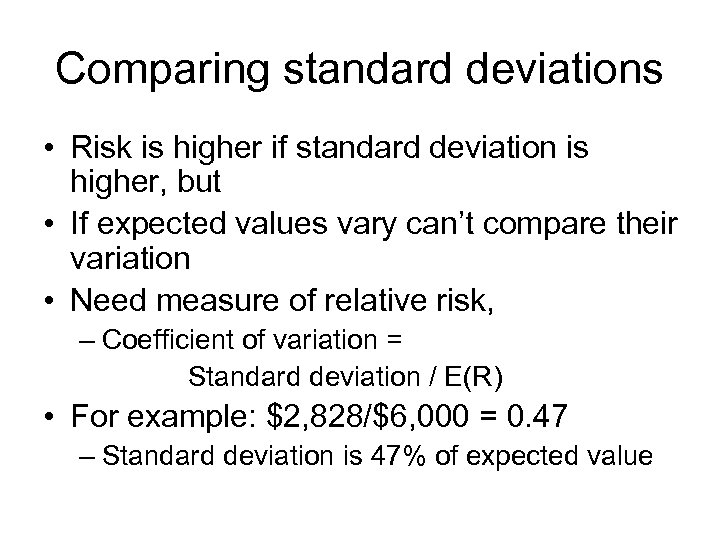Comparing standard deviations • Risk is higher if standard deviation is higher, but • If expected values vary can’t compare their variation • Need measure of relative risk, – Coefficient of variation = Standard deviation / E(R) • For example: \$2, 828/\$6, 000 = 0. 47 – Standard deviation is 47% of expected value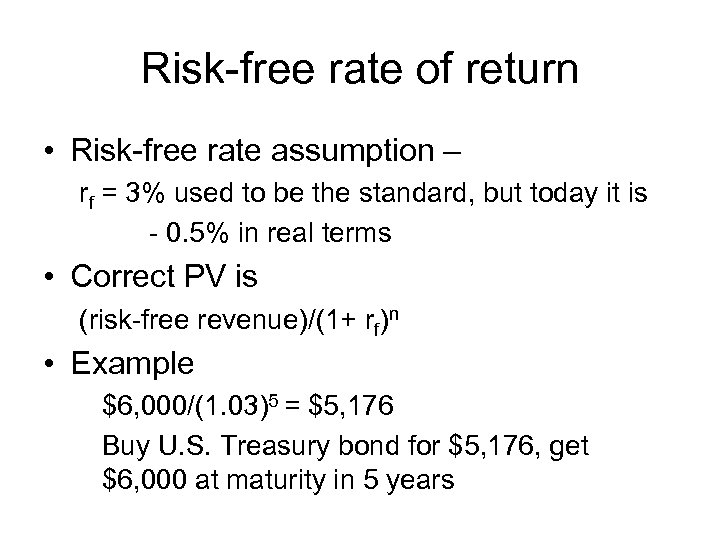Risk-free rate of return • Risk-free rate assumption – rf = 3% used to be the standard, but today it is - 0. 5% in real terms • Correct PV is (risk-free revenue)/(1+ rf)n • Example \$6, 000/(1. 03)5 = \$5, 176 Buy U. S. Treasury bond for \$5, 176, get \$6, 000 at maturity in 5 years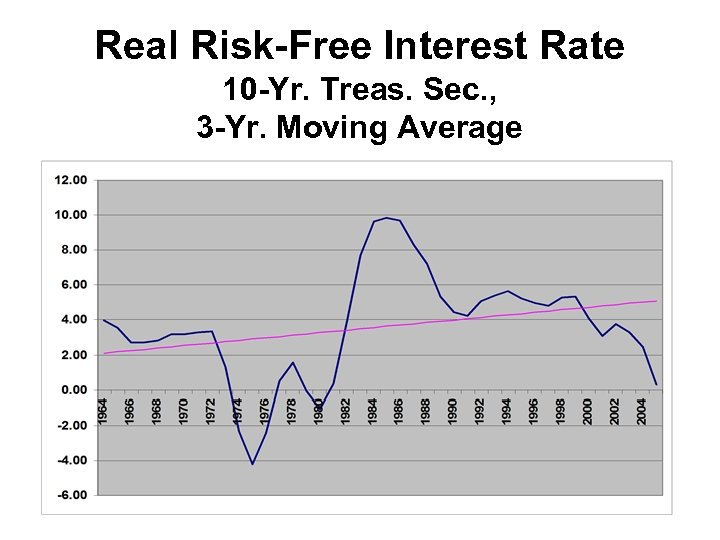Real Risk-Free Interest Rate 10 -Yr. Treas. Sec. , 3 -Yr. Moving Average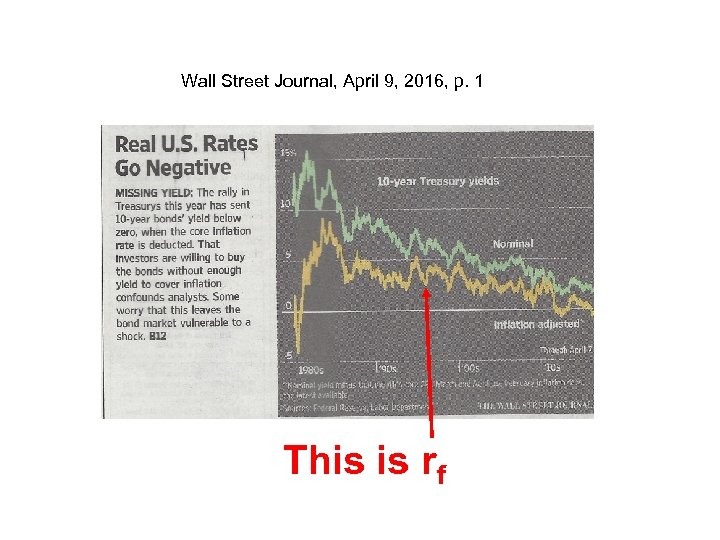Wall Street Journal, April 9, 2016, p. 1 This is rf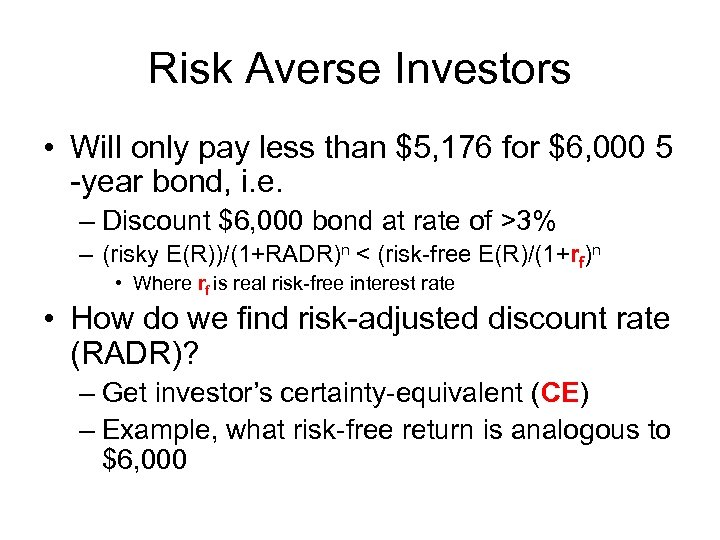Risk Averse Investors • Will only pay less than \$5, 176 for \$6, 000 5 -year bond, i. e. – Discount \$6, 000 bond at rate of >3% – (risky E(R))/(1+RADR)n < (risk-free E(R)/(1+rf)n • Where rf is real risk-free interest rate • How do we find risk-adjusted discount rate (RADR)? – Get investor’s certainty-equivalent (CE) – Example, what risk-free return is analogous to \$6, 000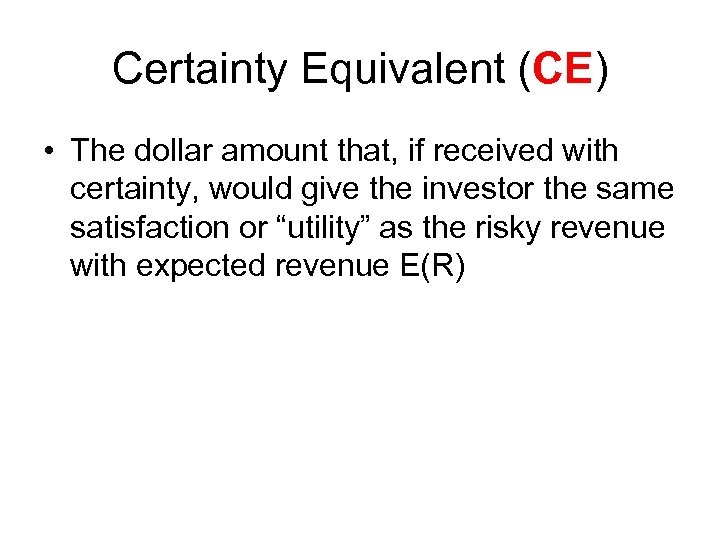Certainty Equivalent (CE) • The dollar amount that, if received with certainty, would give the investor the same satisfaction or “utility” as the risky revenue with expected revenue E(R)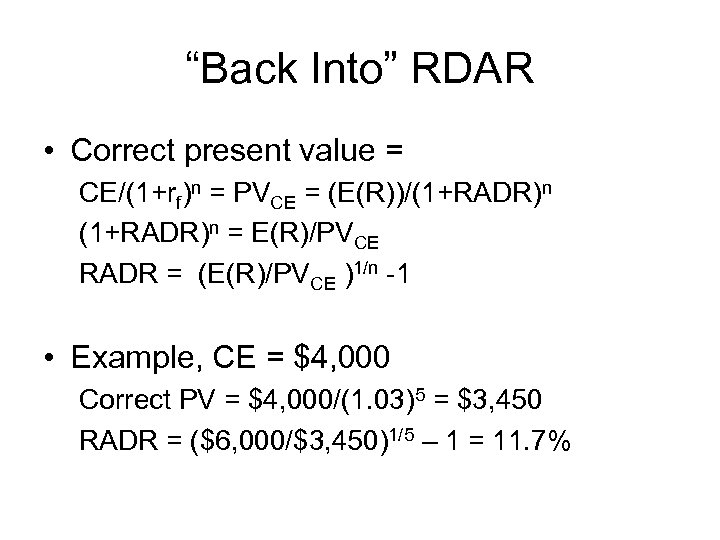“Back Into” RDAR • Correct present value = CE/(1+rf)n = PVCE = (E(R))/(1+RADR)n = E(R)/PVCE RADR = (E(R)/PVCE )1/n -1 • Example, CE = \$4, 000 Correct PV = \$4, 000/(1. 03)5 = \$3, 450 RADR = (\$6, 000/\$3, 450)1/5 – 1 = 11. 7%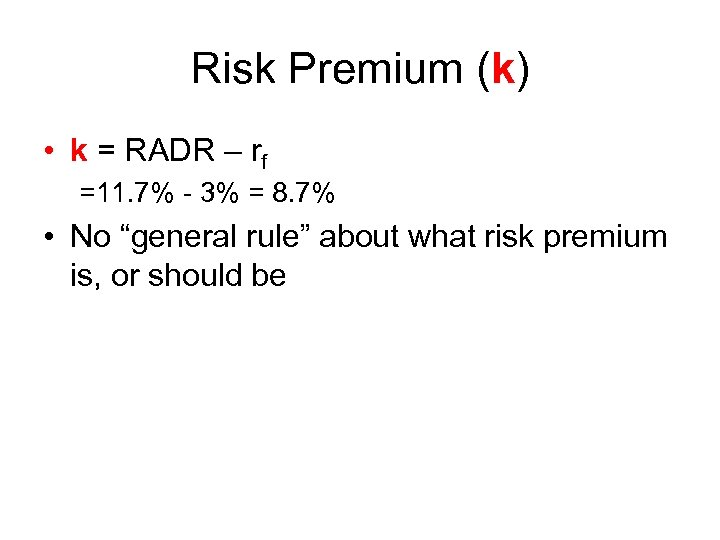Risk Premium (k) • k = RADR – rf =11. 7% - 3% = 8. 7% • No “general rule” about what risk premium is, or should be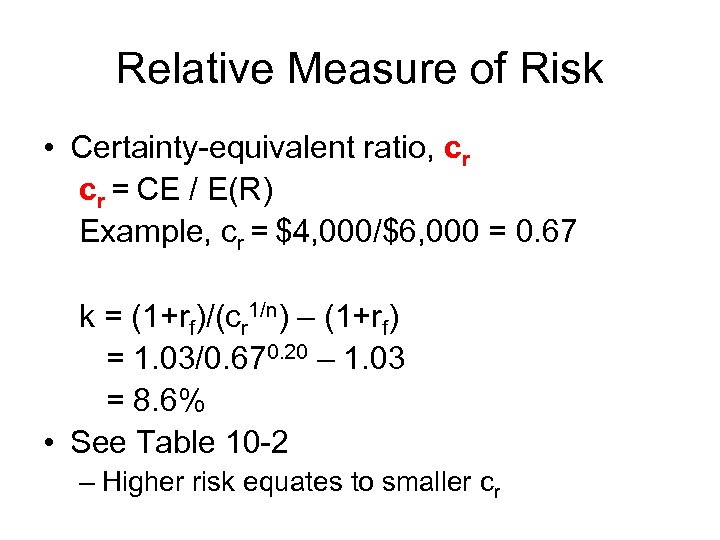Relative Measure of Risk • Certainty-equivalent ratio, cr cr = CE / E(R) Example, cr = \$4, 000/\$6, 000 = 0. 67 k = (1+rf)/(cr 1/n) – (1+rf) = 1. 03/0. 670. 20 – 1. 03 = 8. 6% • See Table 10 -2 – Higher risk equates to smaller cr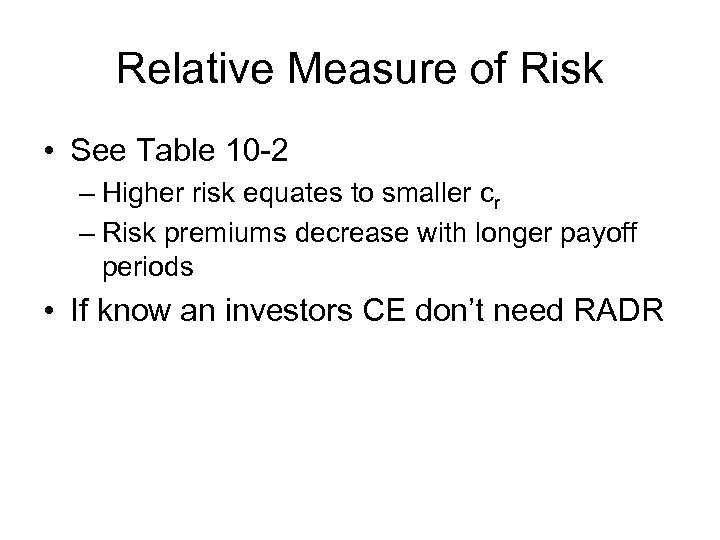Relative Measure of Risk • See Table 10 -2 – Higher risk equates to smaller cr – Risk premiums decrease with longer payoff periods • If know an investors CE don’t need RADR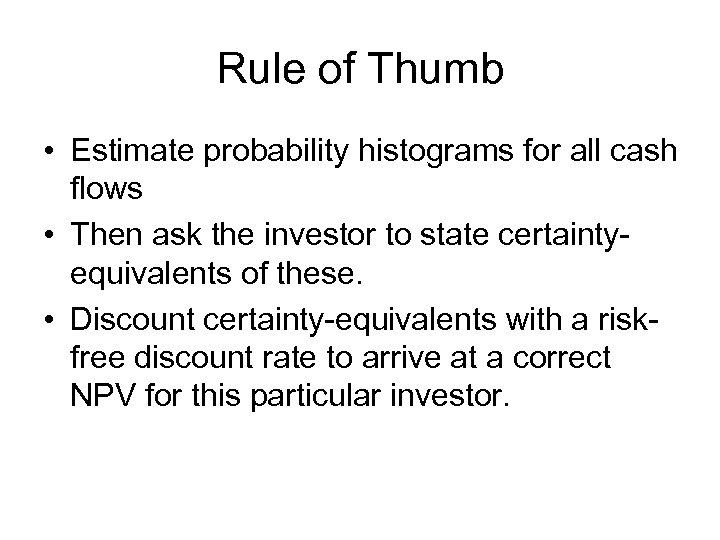Rule of Thumb • Estimate probability histograms for all cash flows • Then ask the investor to state certaintyequivalents of these. • Discount certainty-equivalents with a riskfree discount rate to arrive at a correct NPV for this particular investor.